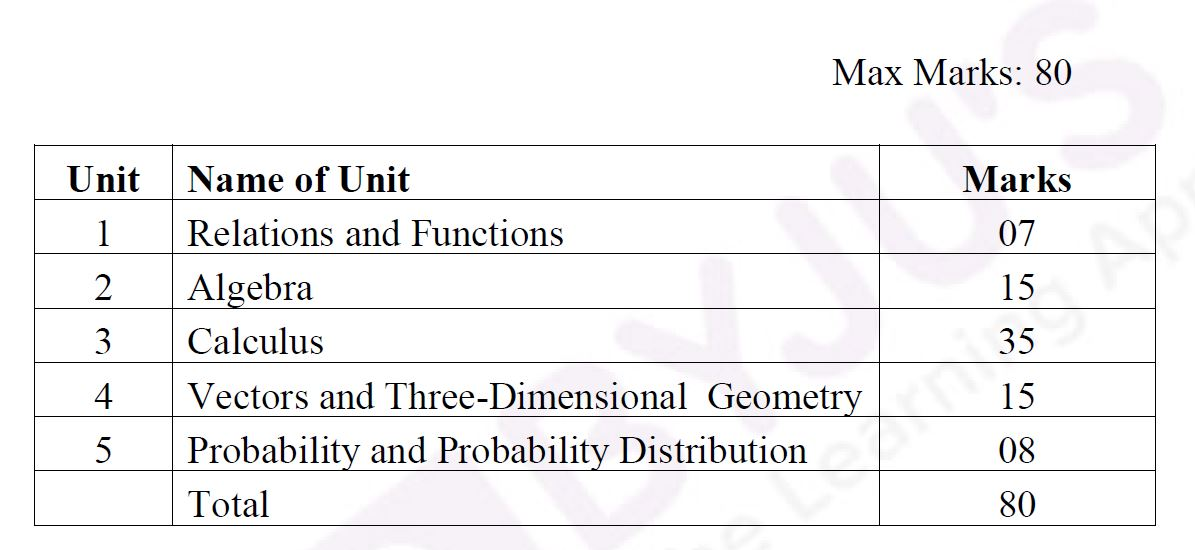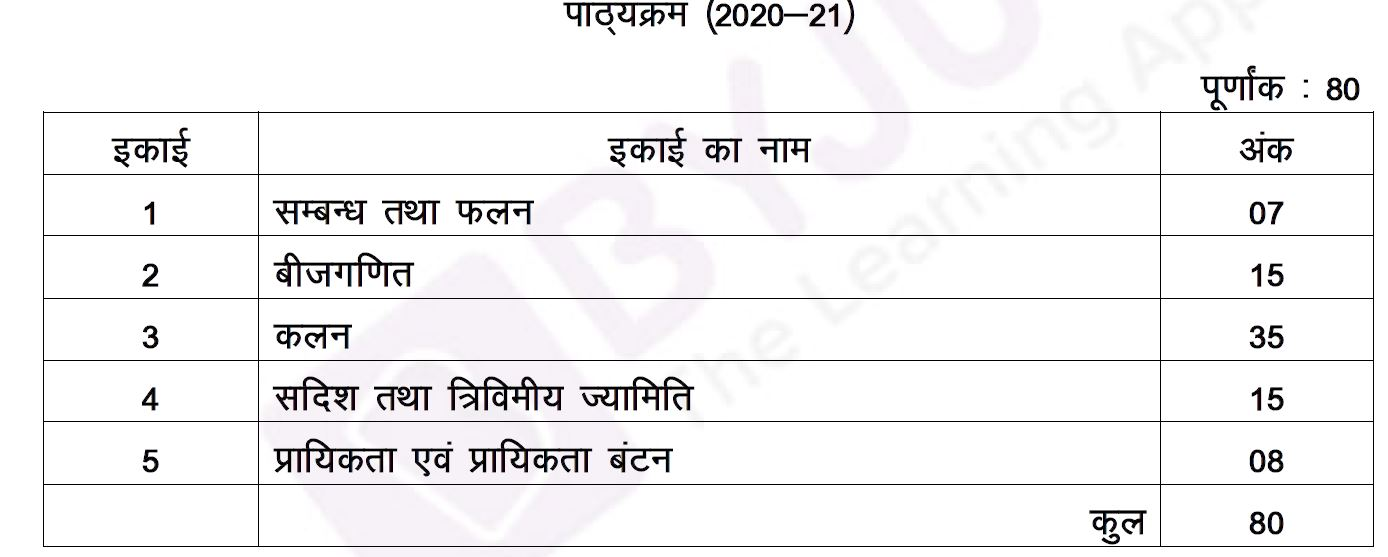# Rajasthan Board Class 12(RBSE Class 12th) Maths Syllabus

RBSE Class 12th Maths Syllabus helps students to know which are the subtopics and concepts being discussed under the subject. If the students understand the syllabus thoroughly, then they can prepare most effectively for the exams. It will help students to grasp the amount of effort required from an examination point of view and plan their academic year accordingly.

Higher mathematics is a fundamental discipline that finds application in various other science subjects. Therefore Rajasthan Board Class 12 Maths Syllabus is designed to include all the topics such as Differential and Integral Calculus to equip the students to face the rigours of higher education. Topics such as Linear Programming and Mathematical Modelling prepares the students for Engineering courses.

## Rajasthan Board Class 12 (RBSE 12th) Maths Syllabus

The Rajasthan Board syllabus for mathematics is divided into 6 units. These units are further subdivided into chapters. Students can have a look at all the chapters of Maths subject from Rajasthan Board Class 12 Textbook. To download the detailed RBSE Class 12 Maths syllabus, visit the link below.

### Download Rajasthan Board Class 12 Revised Maths Syllabus 2020-21 PDF(Hindi Medium)

Students can easily find details of the unit-wise marks weightage from this article. Check the details mentioned.Meanwhile, find also the chapters under each unit of the syllabus below:

Unit I-Composite Function

1. Function part of syllabus deleted

2. Inverse Circular Function

Unit II- Algebra

1. Matrices part of syllabus deleted

2. Determination part of syllabus deleted

3. Inverse Matrix and Linear Equations part of syllabus deleted

Unit III-Calculus

1. Continuity and Differentiability part of syllabus deleted
2. Application of Derivatives  part of syllabus deleted
3. Integration part of syllabus deleted
4. Application of Integral part of syllabus deleted
5. Differential Equations   part of syllabus deleted

Unit IV- Vector and Three Dimensional Geometry

1. Vector part of syllabus deleted
2. Three Dimensional Geometry part of syllabus deleted

Unit V- Linear Programming  Full chapter deleted

Unit VI-Probability and Probability Distribution part of the chapter deleted

### Weightage Distribution of RBSE Class 12 (RBSE Class 12th) Maths Syllabus

The table below shows the unit wise marks distribution of Rajasthan Board syllabus for mathematics subject in Hindi.We hope you find this information on “Rajasthan Board Class 12 Maths Syllabus” useful. Stay tuned for the latest updates on Rajasthan Board Exam. Students can access interactive lessons and videos related to Maths and Science with BYJU’S Tablet/App.At BYJU’S, we are always trying to make learning as much easier as it is possible for the students by providing all the information they need. Obtaining and publishing updated Rajasthan Board Class 12 Maths Syllabus from official sources is a part of this endeavour. After completing the syllabus from the textbook, students must practice the Rajasthan Board class 12th previous years question papers to score good marks in board exams.by India's top teacher with
live doubt solving